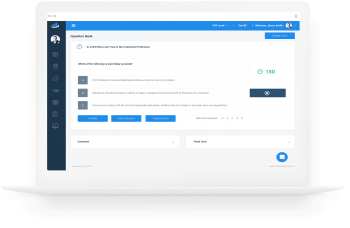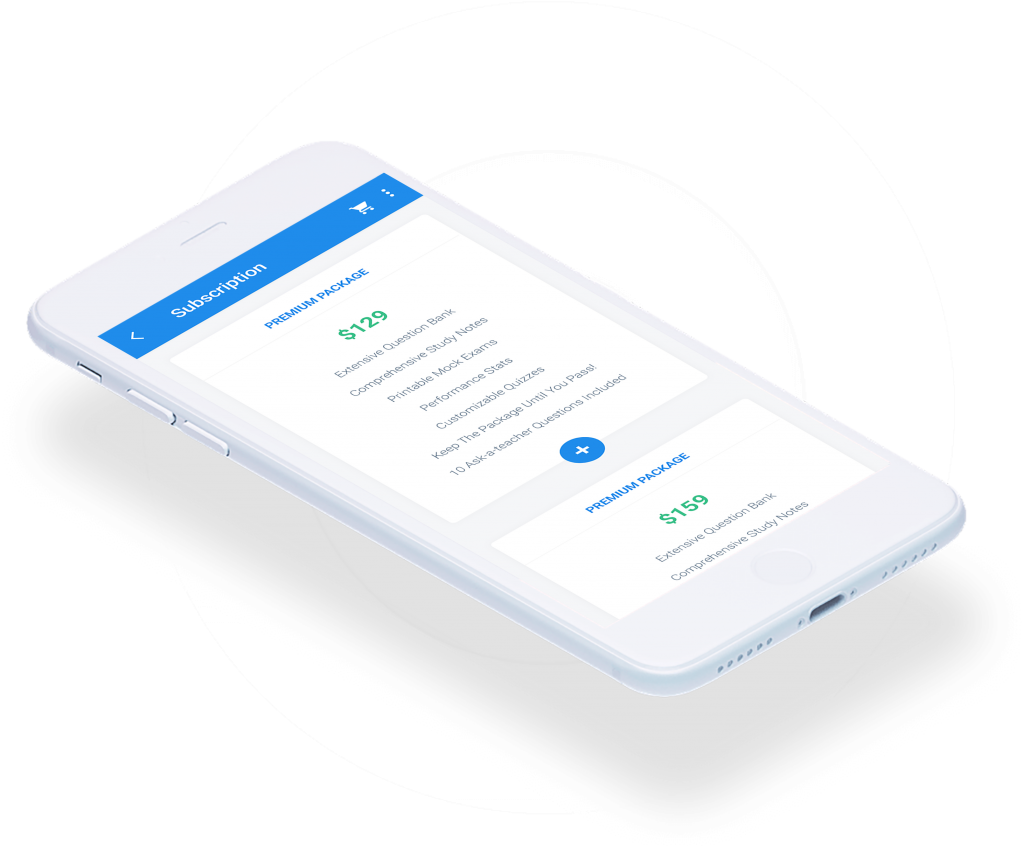Limited Time Offer: Save 10% on all 2021 and 2022 Premium Study Packages with promo code: BLOG10    Select your Premium Package »

Assumptions of the Black-Scholes-Merton Option Valuation Model

The Black-Scholes-Merton (BSM) model is an option pricing model under which the underlying share prices evolve in continuous time and are characterized at any point in time by a continuous distribution rather than a discrete distribution. The following key assumptions…

Expectations Valuation Approach

One-Step Binomial Tree The idea that a hedged portfolio returns the risk-free rate can determine the initial value of a call or put. The expectations approach calculates the values of the option by taking the present value of the expected…

No-Arbitrage Values of Options

Valuing European Options A European option is an option that can only be exercised at expiry. Consider a stock with an initial price of \$70 and a risk-free rate of 1% per year. The asset price can move up by…

Binomial Option Valuation Model

One-Period Binomial Option Valuation Model In the one-period binomial model, we start today (at time t=0) when the stock price is $$S_{0}$$. Then, the stock price can either jump upwards or downwards over the one-period time interval to t=1. This…

Study Notes for CFA® Level II – Derivatives – offered by AnalystPrep

Reading 37: Pricing and Valuation of Forward Commitments -a. Describe and compare how equity, interest rate, fixed-income, and currency forward and futures contracts are priced and valued;  –b. Calculate and interpret the no-arbitrage value of equity, interest rate, fixed-income, and…

Pricing and Valuation of Interest Rate Swaps

Swaps are typically derivative contracts in which two parties exchange (swap) cash flows or other financial instruments over multiple periods for a give-and-take benefit, usually to manage risk. Both swap contract parties have future obligations. Thus, similar to forwards and…

Pricing and Valuation Concepts

A forward commitment is a derivative contract that allows one to buy or sell an underlying security at a predetermined price at a future date. The price of a forward or a futures contract is the prespecified price that the…

Preparation Platform for the Three Levels of the CFA® ExamView More
Recent Posts
FRM® Study PlatformView More
Video Series – Level I of the CFA® Exam